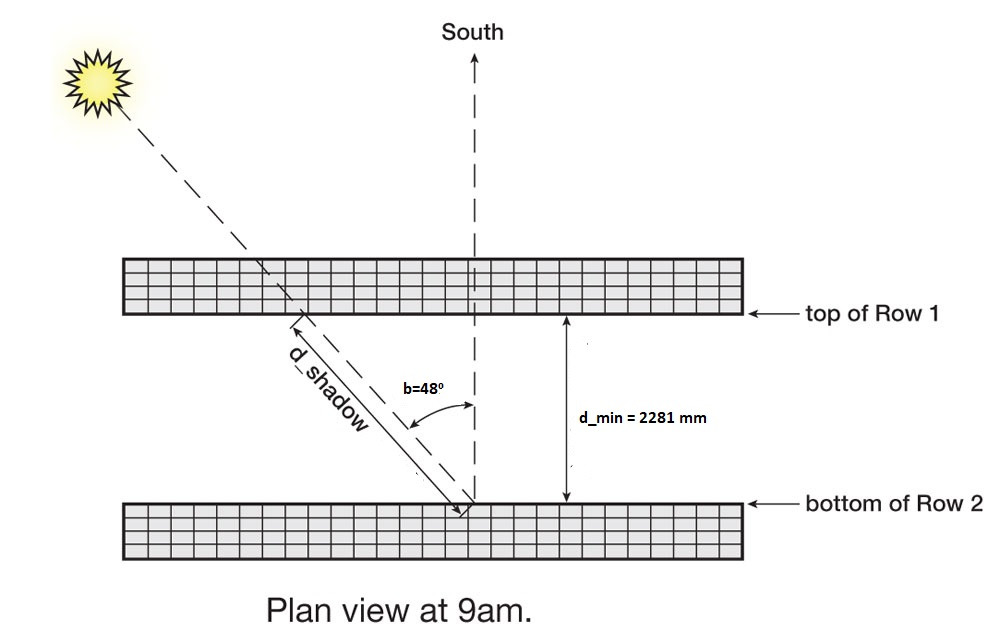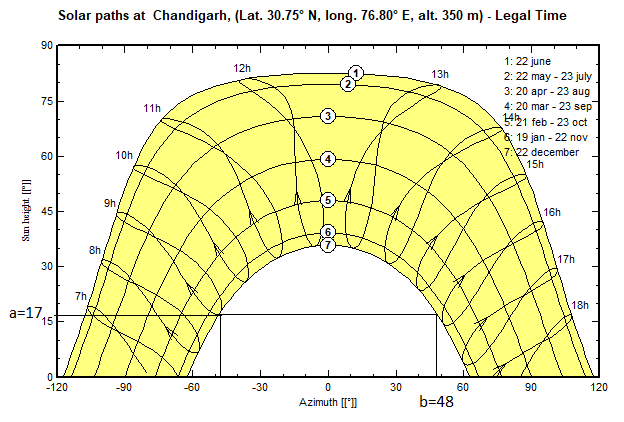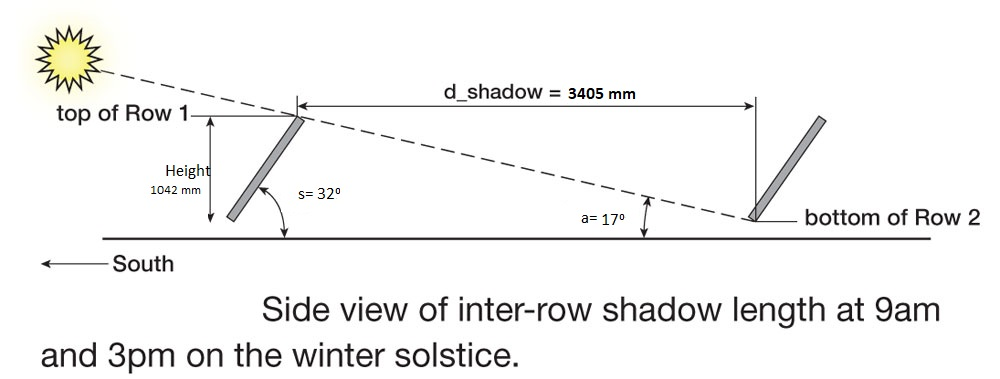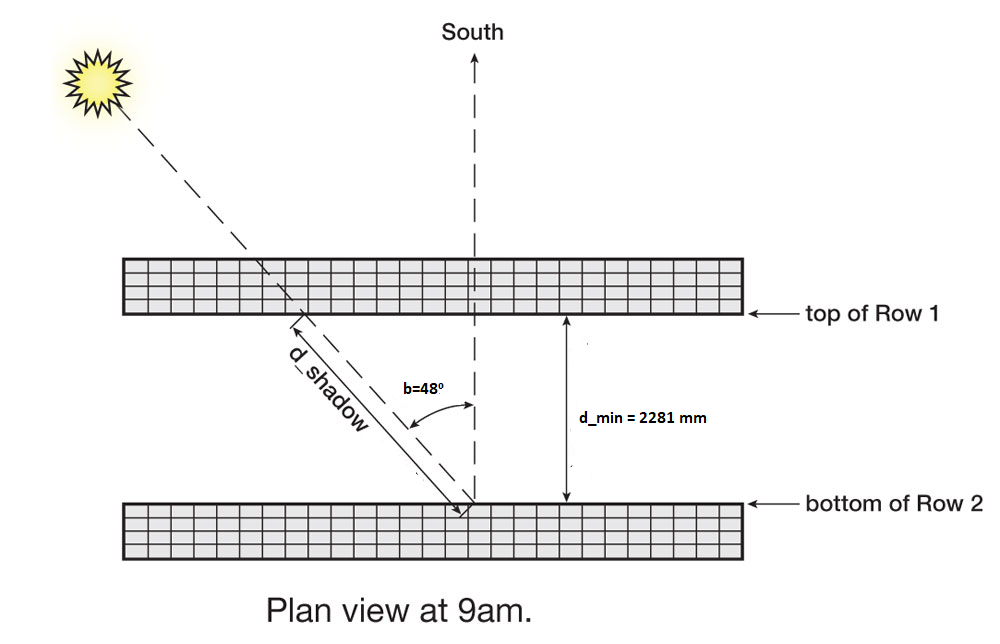Home » Knowledge Center » How to calculate inter-row spacing on ground or flat roof top solar plant

# How to calculate inter-row spacing on ground or flat roof top solar plantCalculating inter row spacing between panel rows is very important. Its creating right balance between maximum power output and maximum area utilizing. Initially we need to calculate sun angle and sun azimuth for maximum working hours of solar system according to your location.This can be picked from sun graph path of your location. I will take Chandigarh as an example for calculations.

Distance is dependent on following parameter :

1. Angle of solar panel –  s
2. Width of solar panel – panel-width
3. Height of panel from top north side – height
4. Working Hours – Morning/Evening
5. Sun angle (According to start time) – a
6. Sun Azimuth (According to start time)  – bMaximum exposure for solar panel is for 9:00 am to 3:40 pm. So we calculate sun angle and sun azimuth for the same. According to graph sun angle (a ) = 17 degree and sun azimuth (b) comes to be 48 degree.

eSun Solar of lots of quality product to choose from. So for example we pick Eging PV 72 Cell Module  . Assuming Panels are placed Vertically. So panel-width = 1966mm.

height = Sin (Tilt Angle) x panel-width

height = sin(32) x 1966 = 0.53 x 1966 = 1042 mm (round off)

from height we can calculate:

d_shadow = Height  / Tan (sun angle)

d_shadow = 1042 / Tan(17) = 1042 / 0.306 = 3405 mmThis is not the minimum distance we need. Since at 9 AM and 3:40 PM sun has a azimuth (b) of 48 degree,minimum will be less.

Calculation for d_min will be as :

d_min = d_shadow x Cos(sun azimuth)

d_min = 3405mm x Cos(48) = 3405 x 0.67 = 2281 mm

d_min = 2281 mmd_min = d_shadow x Cos(sun azimuth)

d_min = Height  / Tan (sun angle) x Cos(sun azimuth)

## d_min =  Sin (Tilt Angle) x panel-width x Cos(sun azimuth)  / Tan (sun angle)